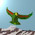## Tuesday, May 18, 2010

### M Theory Lesson 329

There are $27$ possible numerators in the cubic analogue of the two $R_2$ factor probability set. As noted earlier, the CKM matrix approximates this tribimaximal double product for three parameters, set to $(a,b,c)$ $=$ $(-0.231, 24, 0.0035)$. In terms of cubic numerators, the CKM entries take the very simple form:which one may easily verify on an old calculator, remembering to normalise and take square roots. Once again the $(td)$ term is a little higher than expected. The third variable $c$ only enters as the $(ub)$ correction to tribimaximal type mixing. The astute reader will see that this $(ub)$ term destroys the unitarity of the CKM matrix, although unitarity is restored in the full product form.

#### 15 comments:

1.This puts $(td)/(ts)$ at $0.233$, which is a little closer to the current bounds than my last estimate. The SM fit is looking better and better.

2.Actually, the $(ub)$ term is better approximated by $c^2 (a^2 + 1) (b^2 + 1)$ (since $a$ is small this doesn't change things much). I like this better because it is more symmetric in $a$ and $b$, and then if $c = 1$ we get exactly the numerator form for the tribimaximal type operators.

3.Hmm, still can't work out what that 0.231 is. In fact, 25/108 works a bit better. Then we get $0.232$ for $(td)/(ts)$, from $0.00939$.

4.Well done. Instead of normalizing with ts = 1, can you now re-scale all the a, b and c factors to have ts = 0.04062?

5.There is a pattern to these interaction rate factors when drawn in an arrowed diagram. (Remove this comment by all means.)

Nige

6.Dr Sheppeard can I just ask you why you have a minus sign in the "a" term, when it is always appears physically in your table in in the form of a^2, which (aside from the complex conjugate i) turns negative factors into positive ones?

So why not just have a = +0.231, instead of -0.231?

7.OK, Nigel. I was going to get onto the category theory eventually. Anonymous, the minus comes from the original product form fitting. Of course, it doesn't matter much if it is there or not.

8.Re rescaling: anon, note that this choice uses the entries $(td)$, $(cs)$ and $(ub)$ as the 3 parameters. One cannot do better, but perhaps one could make other choices for the quark pairs, such as $(ts)$, $(cb)$ and $(ud)$.

9.For a few weeks I've been studying the F-theory GUT program, mostly for its intrinsic interest, but I've also been waiting to see what happens if you combine it with these new parametrizations of yours, and the algebra behind them. Well, today we have an F-theory paper which talks about quantum tori and the cube root of unity...

10....so this has made me finally try to line up what you're doing against what they're doing.

The F-GUT models don't give exact values for the matrix elements, but there is a model for the orders of magnitude. As described here, the prediction is

1, $\epsilon$, $\epsilon^3$,
$\epsilon$, 1, $\epsilon^2$,
$\epsilon^3$, $\epsilon^2$, 1

which has a decent fit for epsilon = .02, "square root of the GUT fine structure constant", against the actual values

0.97, 0.23, 0.0004,
0.23, 0.97, 0.04,
0.008, 0.04, 0.99

Now to compare this to what you do above. First to list which elements we're talking about:

ud, cd, td,
us, cs, ts,
ub, cb, tb

and finally, listing your polynomials

$(b^2+1)$, $a^2b^2$, $a^2$,
$a^2(b^2+1)$, $b^2$, 1
$c^2(b^2+1)$, $a^2+1$, $b^2(a^2+1)$

Since the $\epsilon$-matrix is just giving powers of $\epsilon$, all we really need is to understand how your abc-quantities produce their hierarchy. Then it becomes clear - b is large, a is smaller, c is very small. Since $\epsilon$ is less than one, they correspond roughly to increasing powers of $\epsilon$.

Since both you and the F-theorists are approximating the same numbers, it was to be expected that there would be at least that much similarity. It remains to be seen whether the deeper algebra has any such resemblance!

11.Grrrrr ... Google have made a mess of blog comments.

Mitchell, we do expect many connections to F theory, especially if they are talking about tori, but I cannot imagine that a GUT constant will ever be a more physical way of looking at this than QI/categories, since it is so elegant and easy to understand.

Then again, I am glad that you are doing this, Mitchell. Perhaps you might convince one or two string theorists that pure QI is closely connected to what they are trying to do in understanding M/F theory using rough QI + holography.

12.Wow, Mitchell! I just read Lubos' Fuzzy F Theory post, and it seems that they really are talking about the 24. Do you think Vafa will give me a job?

13.Ah, that might be it: $25/108$ is equal to $(25/24)(2/9)$. At least that would bring the second parameter into line with a lepton parameter.

14.I see now that you already mentioned Vafa's matrix last year.

15.Ah, no. After looking at MNS data, it seems that a better guess would be $a = -0.2322$ (which still gives a good fit) because this is just $\sqrt{2} /6 - c$.

Note: Only a member of this blog may post a comment.